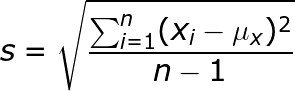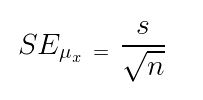# Program to implement standard error of mean

Standard error of mean (SEM) is used to estimate the sample mean dispersion from the population mean. The standard error along with sample mean is used to estimate the approximate confidence intervals for the mean. It is also known as standard error of mean or measurement often denoted by SE, SEM or SE.

Examples:

```Input : arr[] = {78.53, 79.62, 80.25, 81.05, 83.21, 83.46}
Output : 0.8063

Input : arr[] = {5, 5.5, 4.9, 4.85, 5.25, 5.05, 6.0}
Output : 0.1546
```

Sample meanSample Standard DeviationEstimate standard error of meanExplanation:

given an array arr[] = {78.53, 79.62, 80.25, 81.05, 83.21, 83.46}
and the task is to find standard error of mean.
mean = (78.53 + 79.62 + 80.25 + 81.05 + 83.21 + 83.46) / 6
= 486.12 / 6
= 81.02
Sample Standard deviation = sqrt((78.53 – 81.02)2 + (79.62- 81.02)2 + . . .
+ (83.46 – 81.02)2 / (6 – 1))
= sqrt(19.5036 / 5)
= 1.97502
Standard error of mean = 1.97502 / sqrt(6)
= 0.8063

## C++

 `// C++ Program to implement  ` `// standard error of mean. ` `#include ` `using` `namespace` `std; ` ` `  `// Function to find sample mean. ` `float` `mean(``float` `arr[], ``int` `n) ` `{    ` `    ``// loop to calculate  ` `    ``// sum of array elements. ` `    ``float` `sum = 0; ` `    ``for` `(``int` `i = 0; i < n; i++) ` `        ``sum = sum + arr[i]; ` `     `  `    ``return` `sum / n; ` `} ` ` `  `// Function to calculate sample ` `// standard deviation. ` `float` `SSD(``float` `arr[], ``int` `n) ` `{ ` `    ``float` `sum = 0;     ` `    ``for` `(``int` `i = 0; i < n; i++) ` `        ``sum = sum + (arr[i] - mean(arr, n)) ` `                    ``* (arr[i] - mean(arr, n)); ` ` `  `    ``return` `sqrt``(sum / (n - 1)); ` `} ` ` `  ` `  `// Function to calculate sample error. ` `float` `sampleError(``float` `arr[], ``int` `n) ` `{     ` `    ``// Formula to find sample error. ` `    ``return` `SSD(arr, n) / ``sqrt``(n); ` `} ` ` `  `// Driver function ` `int` `main() ` `{ ` `    ``float` `arr[] = { 78.53, 79.62, 80.25, ` `                    ``81.05, 83.21, 83.46 }; ` `    ``int` `n = ``sizeof``(arr) / ``sizeof``(arr); ` `    ``cout << sampleError(arr, n);     ` `    ``return` `0; ` `} `

## Java

 `// Java Program to implement ` `// standard error of mean. ` ` `  `class` `GFG { ` ` `  `    ``// Function to find sample mean. ` `    ``static` `float` `mean(``float` `arr[], ``int` `n) ` `    ``{ ` `        ``// loop to calculate ` `        ``// sum of array elements. ` `        ``float` `sum = ``0``; ` `        ``for` `(``int` `i = ``0``; i < n; i++) ` `            ``sum = sum + arr[i]; ` ` `  `        ``return` `sum / n; ` `    ``} ` ` `  `    ``// Function to calculate sample ` `    ``// standard deviation. ` `    ``static` `float` `SSD(``float` `arr[], ``int` `n) ` `    ``{ ` `        ``float` `sum = ``0``; ` `        ``for` `(``int` `i = ``0``; i < n; i++) ` `            ``sum = sum + (arr[i] - mean(arr, n))  ` `                  ``* (arr[i] - mean(arr, n)); ` ` `  `        ``return` `(``float``)Math.sqrt(sum / (n - ``1``)); ` `    ``} ` ` `  `    ``// Function to calculate sample error. ` `    ``static` `float` `sampleError(``float` `arr[], ``int` `n) ` `    ``{ ` `        ``// Formula to find sample error. ` `        ``return` `SSD(arr, n) / (``float``)Math.sqrt(n); ` `    ``} ` ` `  `    ``// Driver function ` `    ``public` `static` `void` `main(String[] args) ` `    ``{ ` `        ``float` `arr[] = { ``78``.53f, ``79``.62f, ``80``.25f,  ` `                       ``81``.05f, ``83``.21f, ``83``.46f }; ` `        ``int` `n = arr.length; ` `        ``System.out.println(sampleError(arr, n)); ` `    ``} ` `} ` ` `  `// This code is contributed  ` `// by  prerna saini `

## Python3

 `# Python 3 Program to implement  ` `# standard error of mean. ` `import` `math ` ` `  ` `  `# Function to find sample mean. ` `def` `mean(arr, n) : ` ` `  `    ``# loop to calculate  ` `    ``# sum of array elements. ` `    ``sm ``=` `0` `    ``for` `i ``in` `range``(``0``,n) : ` `        ``sm ``=` `sm ``+` `arr[i] ` `      `  `    ``return` `sm ``/` `n ` ` `  ` `  `# Function to calculate sample ` `# standard deviation. ` `def` `SSD(arr, n) : ` `    ``sm ``=` `0` `    ``for` `i ``in` `range``(``0``,n) : ` `        ``sm ``=` `sm ``+` `(arr[i] ``-` `mean(arr, n)) ``*` `(arr[i] ``-` `mean(arr, n)) ` `  `  `    ``return` `(math.sqrt(sm ``/` `(n ``-` `1``))) ` `  `  `  `  `# Function to calculate sample error. ` `def` `sampleError(arr, n) : ` ` `  `    ``# Formula to find sample error. ` `    ``return` `SSD(arr, n) ``/` `(math.sqrt(n)) ` ` `  `  `  `# Driver function ` `arr ``=` `[ ``78.53``, ``79.62``, ``80.25``, ``81.05``, ``83.21``, ``83.46``] ` `n ``=` `len``(arr) ` `print``(sampleError(arr, n)) ` `     `  `   `  `# This code is contributed ` `# by Nikita Tiwari. `

## C#

 `// C# Program to implement ` `// standard error of mean. ` `using` `System; ` ` `  `class` `GFG { ` ` `  `    ``// Function to find sample mean. ` `    ``static` `float` `mean(``float` `[]arr, ``int` `n) ` `    ``{ ` `         `  `        ``// loop to calculate ` `        ``// sum of array elements. ` `        ``float` `sum = 0; ` `        ``for` `(``int` `i = 0; i < n; i++) ` `            ``sum = sum + arr[i]; ` ` `  `        ``return` `sum / n; ` `    ``} ` ` `  `    ``// Function to calculate sample ` `    ``// standard deviation. ` `    ``static` `float` `SSD(``float` `[]arr, ``int` `n) ` `    ``{ ` `        ``float` `sum = 0; ` `        ``for` `(``int` `i = 0; i < n; i++) ` `            ``sum = sum + (arr[i] - mean(arr, n))  ` `                      ``* (arr[i] - mean(arr, n)); ` ` `  `        ``return` `(``float``)Math.Sqrt(sum / (n - 1)); ` `    ``} ` ` `  `    ``// Function to calculate sample error. ` `    ``static` `float` `sampleError(``float` `[]arr, ``int` `n) ` `    ``{ ` `         `  `        ``// Formula to find sample error. ` `        ``return` `SSD(arr, n) / (``float``)Math.Sqrt(n); ` `    ``} ` ` `  `    ``// Driver code ` `    ``public` `static` `void` `Main() ` `    ``{ ` `        ``float` `[]arr = {78.53f, 79.62f, 80.25f,  ` `                       ``81.05f, 83.21f, 83.46f}; ` `        ``int` `n = arr.Length; ` `        ``Console.Write(sampleError(arr, n)); ` `    ``} ` `} ` ` `  `// This code is contributed by Nitin Mittal. `

## PHP

 ` `

Output:

```0.8063
```

Attention reader! Don’t stop learning now. Get hold of all the important DSA concepts with the DSA Self Paced Course at a student-friendly price and become industry ready.

My Personal Notes arrow_drop_upCheck out this Author's contributed articles.

If you like GeeksforGeeks and would like to contribute, you can also write an article using contribute.geeksforgeeks.org or mail your article to contribute@geeksforgeeks.org. See your article appearing on the GeeksforGeeks main page and help other Geeks.

Please Improve this article if you find anything incorrect by clicking on the "Improve Article" button below.

Improved By : nitin mittal

Article Tags :
Practice Tags :

Be the First to upvote.

Please write to us at contribute@geeksforgeeks.org to report any issue with the above content.